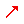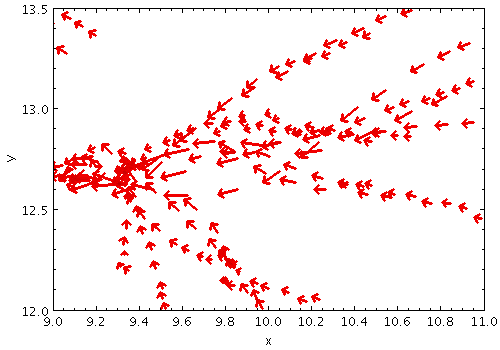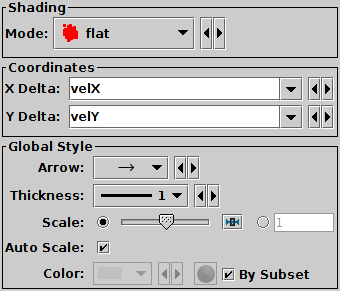Next Previous Up Contents
Next: SkyVector Form
Up: Plot Forms
Previous: SizeXY Form

#### A.4.5.4 Vector Form

The Vector form () plots directed lines from the data position given delta values for the coordinates. The plotted markers are typically little arrows, but there are other options.

In some cases such delta values may be the actual magnitude required for the plot, but often the vector data represents a value which has a different magnitude or is in different units to the positional data. As a convenience for this case, the plotter can optionally scale the magnitudes of all the vectors to make them a sensible size (so the largest ones are a few tens of pixels long). This scaling can be adjusted or turned off using the Scale and Auto Scale options below.Example XYVector plotVector form configuration panel

The configuration options are:

See Appendix A.4.6.
X Delta, Y Delta, ...
The coordinates of the changes in each coordinate which gives the vector. The coordinates here match the coordinates of the plot.
Arrow
Arrow shape selected from a range of options.
Thickness
Controls the line thickness used when drawing arrows. Zero, the default value, means a 1-pixel-wide line is used, and larger values make drawn lines thicker.
Scale
Changes the factor by which all vector sizes are scaled. If the arrows are too small, slide it right, if they are too big, slide it left. The slider scale is logarithmic. Alternatively, enter a fixed value in the text field.
Auto Scale
If selected, this option will determine the default arrow scale size from the data - it will fix it so that the largest arrows are a few tens of pixels long by default. That scaling can then be adjusted using the Scale slider. If unselected, then the default position of the Scale slider corresponds to the actual positions given by the submitted delta coordinates.

Next Previous Up Contents
Next: SkyVector Form
Up: Plot Forms
Previous: SizeXY Form

TOPCAT - Tool for OPerations on Catalogues And Tables